# Final exam Exercises Final Exam Exercises 1 Which

• Slides: 43Final exam ExercisesFinal Exam/ Exercises 1 -Which atom of these following atoms will gain two electrons when forming ion. a-Mg b- S c- Br d- Al 2 -alkali metal from period 4 is: a- Na b- Ca c- K d- Li 3 -The number of kilograms in one teragram are : a-1 x 1015 Kg b- 1 x 109 Kg c- 1 x 10 -9 Kg d- 1 x 10 -15 Kg First comvert from Tg to g : 1 X 1012 m Then from g to Kg 1 x 1012 x 10 -3 = 1 x 109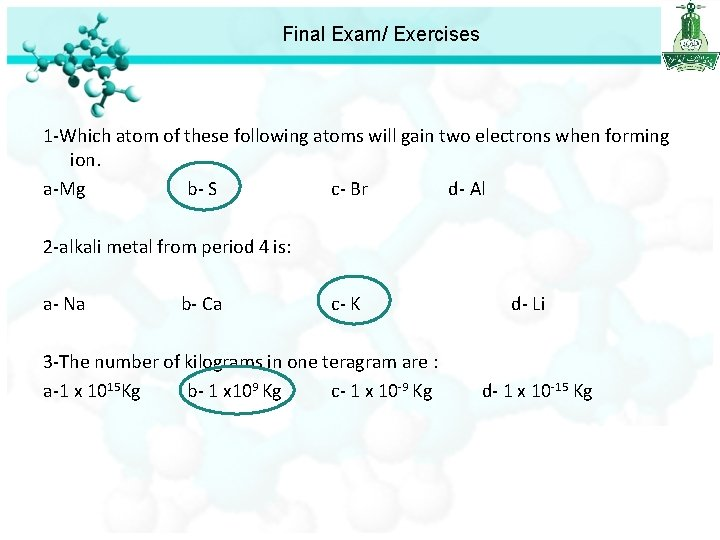Final Exam/ Exercises 1 -Which atom of these following atoms will gain two electrons when forming ion. a-Mg b- S c- Br d- Al 2 -alkali metal from period 4 is: a- Na b- Ca c- K d- Li 3 -The number of kilograms in one teragram are : a-1 x 1015 Kg b- 1 x 109 Kg c- 1 x 10 -9 Kg d- 1 x 10 -15 Kg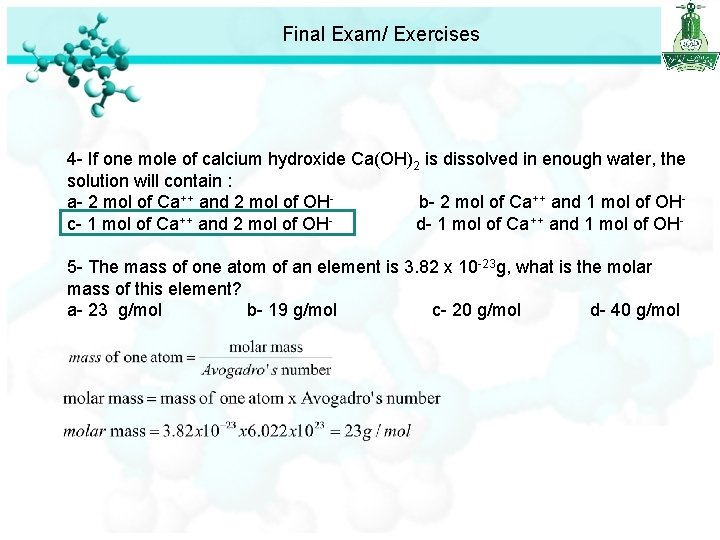Final Exam/ Exercises 4 - If one mole of calcium hydroxide Ca(OH)2 is dissolved in enough water, the solution will contain : a- 2 mol of Ca++ and 2 mol of OHb- 2 mol of Ca++ and 1 mol of OHc- 1 mol of Ca++ and 2 mol of OHd- 1 mol of Ca++ and 1 mol of OH 5 - The mass of one atom of an element is 3. 82 x 10 -23 g, what is the molar mass of this element? a- 23 g/mol b- 19 g/mol c- 20 g/mol d- 40 g/mol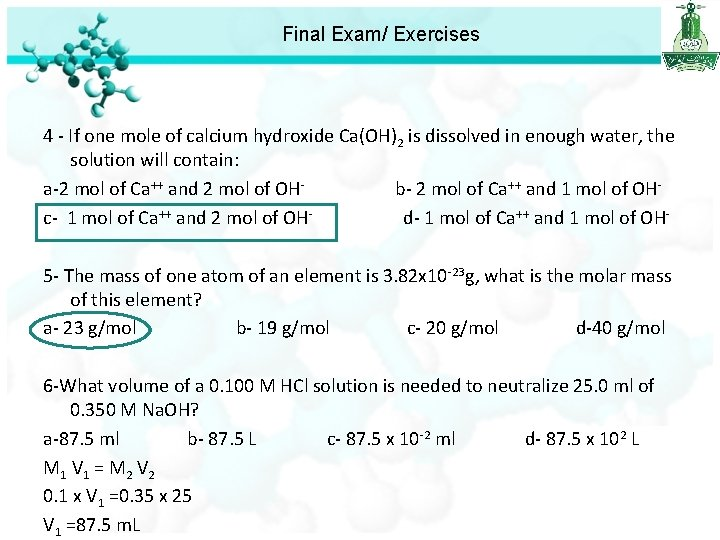Final Exam/ Exercises 4 - If one mole of calcium hydroxide Ca(OH)2 is dissolved in enough water, the solution will contain: a-2 mol of Ca++ and 2 mol of OH- b- 2 mol of Ca++ and 1 mol of OH- c- 1 mol of Ca++ and 2 mol of OH- d- 1 mol of Ca++ and 1 mol of OH 5 - The mass of one atom of an element is 3. 82 x 10 -23 g, what is the molar mass of this element? a- 23 g/mol b- 19 g/mol c- 20 g/mol d-40 g/mol 6 -What volume of a 0. 100 M HCl solution is needed to neutralize 25. 0 ml of 0. 350 M Na. OH? a-87. 5 ml b- 87. 5 L c- 87. 5 x 10 -2 ml d- 87. 5 x 102 L M 1 V 1 = M 2 V 2 0. 1 x V 1 =0. 35 x 25 V 1 =87. 5 m. L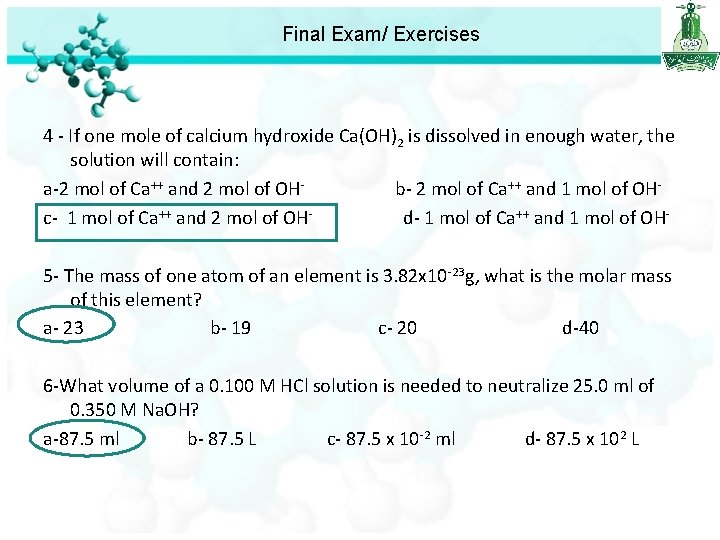Final Exam/ Exercises 4 - If one mole of calcium hydroxide Ca(OH)2 is dissolved in enough water, the solution will contain: a-2 mol of Ca++ and 2 mol of OH- b- 2 mol of Ca++ and 1 mol of OH- c- 1 mol of Ca++ and 2 mol of OH- d- 1 mol of Ca++ and 1 mol of OH 5 - The mass of one atom of an element is 3. 82 x 10 -23 g, what is the molar mass of this element? a- 23 b- 19 c- 20 d-40 6 -What volume of a 0. 100 M HCl solution is needed to neutralize 25. 0 ml of 0. 350 M Na. OH? a-87. 5 ml b- 87. 5 L c- 87. 5 x 10 -2 ml d- 87. 5 x 102 L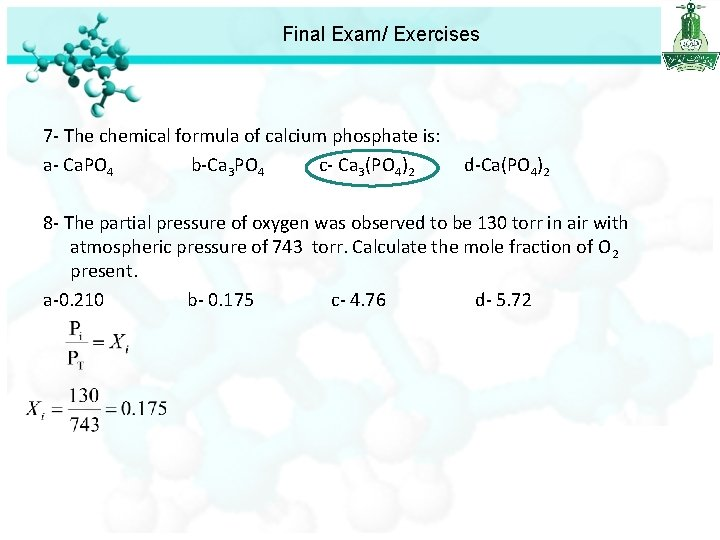Final Exam/ Exercises 7 - The chemical formula of calcium phosphate is: a- Ca. PO 4 b-Ca 3 PO 4 c- Ca 3(PO 4)2 d-Ca(PO 4)2 8 - The partial pressure of oxygen was observed to be 130 torr in air with atmospheric pressure of 743 torr. Calculate the mole fraction of O 2 present. a-0. 210 b- 0. 175 c- 4. 76 d- 5. 72Final Exam/ Exercises 7 - The chemical formula of calcium phosphate is: a- Ca. PO 4 b-Ca 3 PO 4 c- Ca 3(PO 4)2 d-Ca(PO 4)2 8 - The partial pressure of oxygen was observed to be 130 torr in air with atmospheric pressure of 743 torr. Calculate the mole fraction of O 2 present. a-0. 210 b- 0. 175 c- 4. 76 d- 5. 72 9 - In a process 500 g of Na. Cl are treated with an excess of H 2 SO 4 and yield 500 g of Na 2 SO 4. Calculate the percent yield of Na 2 SO 4. 2 Na. Cl + H 2 SO 4 Na 2 SO 4 + 2 HCl a- 77. 51% b- 90. 7% c- 82. 39% d- 85. 76%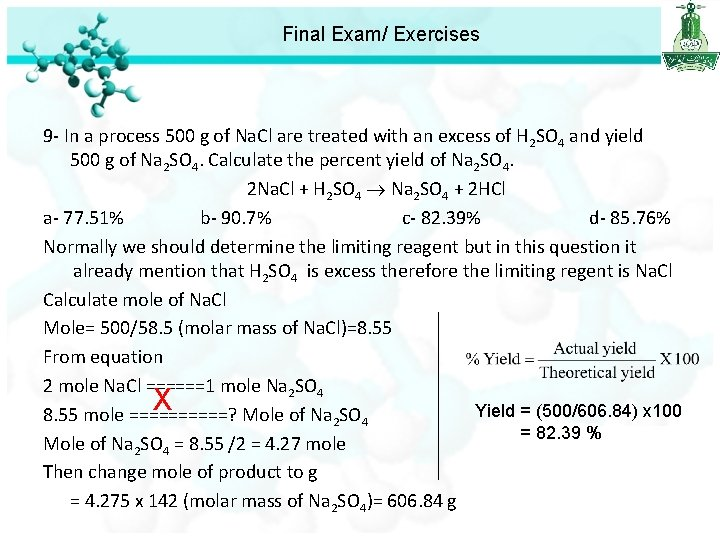Final Exam/ Exercises 9 - In a process 500 g of Na. Cl are treated with an excess of H 2 SO 4 and yield 500 g of Na 2 SO 4. Calculate the percent yield of Na 2 SO 4. 2 Na. Cl + H 2 SO 4 Na 2 SO 4 + 2 HCl a- 77. 51% b- 90. 7% c- 82. 39% d- 85. 76% Normally we should determine the limiting reagent but in this question it already mention that H 2 SO 4 is excess therefore the limiting regent is Na. Cl Calculate mole of Na. Cl Mole= 500/58. 5 (molar mass of Na. Cl)=8. 55 From equation 2 mole Na. Cl ======1 mole Na 2 SO 4 X Yield = (500/606. 84) x 100 8. 55 mole =====? Mole of Na 2 SO 4 = 82. 39 % Mole of Na 2 SO 4 = 8. 55 /2 = 4. 27 mole Then change mole of product to g = 4. 275 x 142 (molar mass of Na 2 SO 4)= 606. 84 gFinal Exam/ Exercises 7 - The chemical formula of calcium phosphate is: a- Ca. PO 4 b-Ca 3 PO 4 c- Ca 3(PO 4)2 d-Ca(PO 4)2 8 - The partial pressure of oxygen was observed to be 130 torr in air with atmospheric pressure of 743 torr. Calculate the mole fraction of O 2 present. a-0. 210 b- 0. 175 c- 4. 76 d- 5. 72 9 - In a process 500 g of Na. Cl are treated with an excess of H 2 SO 4 and yield 500 g of Na 2 SO 4. Calculate the percent yield of Na 2 SO 4. 2 Na. Cl + H 2 SO 4 Na 2 SO 4 + 2 HCl a- 77. 51% b- 90. 7% c- 82. 39% d- 85. 76%Final Exam/ Exercises 10 - The appropriate symbol for an element with Z = 45 and A = 103 is : a-Rh b- Sc c- Lr d- Rn 11 - What is the molecular weight of Cu. SO 4· 5 H 2 O? a-249. 5 gmol-1 b- 177. 5 gmol-1 c- 127 gmol-1 d- 87 gmol-1 12 - How many carbon (C) atoms are present in 2 g of nitroglycerin C 3 H 5 N 3 O 9? a-1. 59 x 1022 atom b- 2. 65 x 1022 atom c- 4. 78 x 1022 atom d- none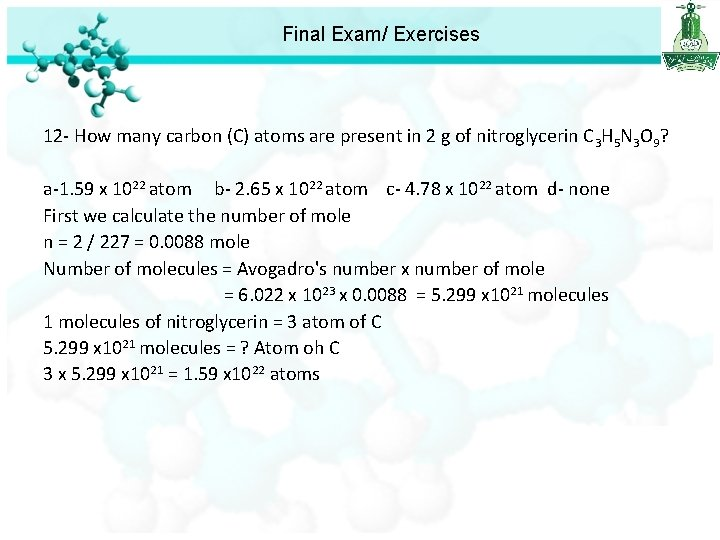Final Exam/ Exercises 12 - How many carbon (C) atoms are present in 2 g of nitroglycerin C 3 H 5 N 3 O 9? a-1. 59 x 1022 atom b- 2. 65 x 1022 atom c- 4. 78 x 1022 atom d- none First we calculate the number of mole n = 2 / 227 = 0. 0088 mole Number of molecules = Avogadro's number x number of mole = 6. 022 x 1023 x 0. 0088 = 5. 299 x 1021 molecules of nitroglycerin = 3 atom of C 5. 299 x 1021 molecules = ? Atom oh C 3 x 5. 299 x 1021 = 1. 59 x 1022 atoms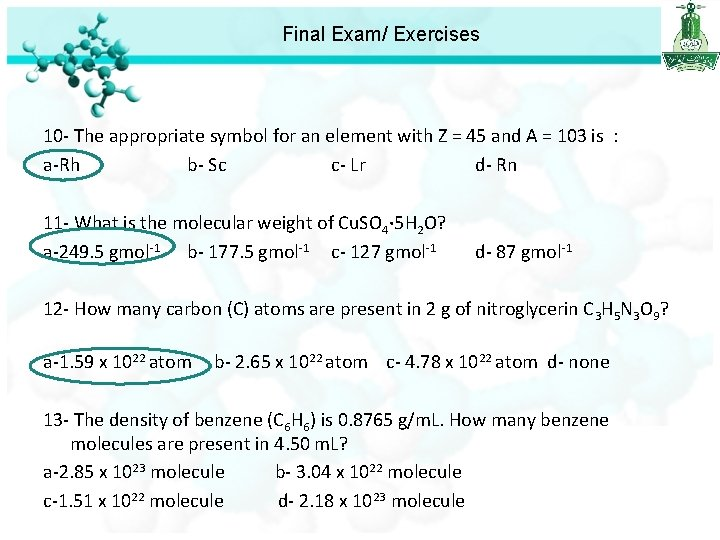Final Exam/ Exercises 10 - The appropriate symbol for an element with Z = 45 and A = 103 is : a-Rh b- Sc c- Lr d- Rn 11 - What is the molecular weight of Cu. SO 4· 5 H 2 O? a-249. 5 gmol-1 b- 177. 5 gmol-1 c- 127 gmol-1 d- 87 gmol-1 12 - How many carbon (C) atoms are present in 2 g of nitroglycerin C 3 H 5 N 3 O 9? a-1. 59 x 1022 atom b- 2. 65 x 1022 atom c- 4. 78 x 1022 atom d- none 13 - The density of benzene (C 6 H 6) is 0. 8765 g/m. L. How many benzene molecules are present in 4. 50 m. L? a-2. 85 x 1023 molecule b- 3. 04 x 1022 molecule c-1. 51 x 1022 molecule d- 2. 18 x 1023 molecule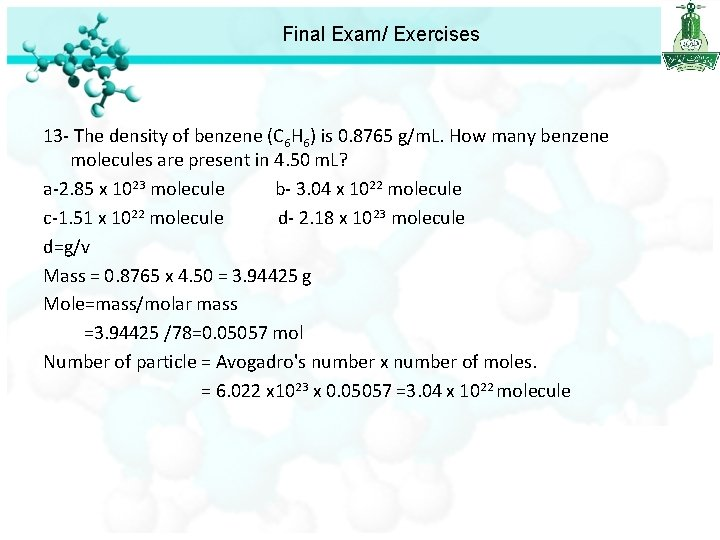Final Exam/ Exercises 13 - The density of benzene (C 6 H 6) is 0. 8765 g/m. L. How many benzene molecules are present in 4. 50 m. L? a-2. 85 x 1023 molecule b- 3. 04 x 1022 molecule c-1. 51 x 1022 molecule d- 2. 18 x 1023 molecule d=g/v Mass = 0. 8765 x 4. 50 = 3. 94425 g Mole=mass/molar mass =3. 94425 /78=0. 05057 mol Number of particle = Avogadro's number x number of moles. = 6. 022 x 1023 x 0. 05057 =3. 04 x 1022 moleculeFinal Exam/ Exercises 10 - The appropriate symbol for an element with Z = 45 and A = 103 is : a-Rh b- Sc c- Lr d- Rn 11 - What is the molecular weight of Cu. SO 4· 5 H 2 O? a-249. 5 gmol-1 b- 177. 5 gmol-1 c- 127 gmol-1 d- 87 gmol-1 12 - How many carbon (C) atoms are present in 2 g of nitroglycerin C 3 H 5 N 3 O 9? a-1. 59 x 1022 atom b- 2. 65 x 1022 atom c- 4. 78 x 1022 atom d- none 13 - The density of benzene (C 6 H 6) is 0. 8765 g/m. L. How many benzene molecules are present in 4. 50 m. L? a-2. 85 x 1023 molecule b- 3. 04 x 1022 molecule c-1. 51 x 1022 molecule d- 2. 18 x 1023 molecule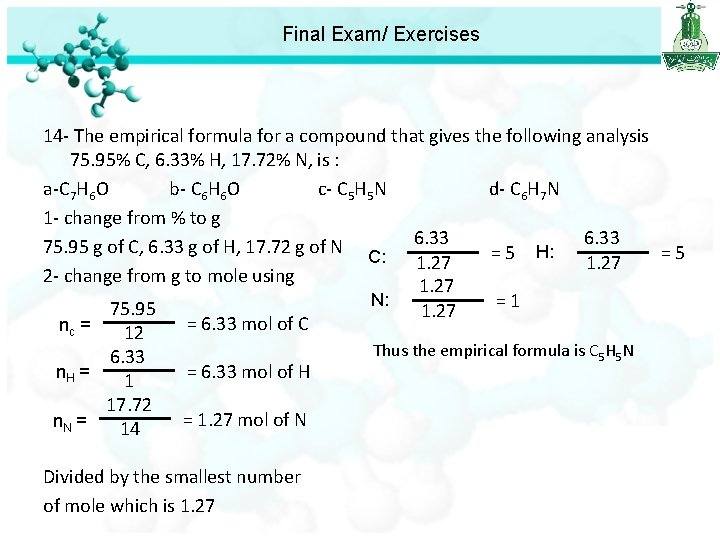Final Exam/ Exercises 14 - The empirical formula for a compound that gives the following analysis 75. 95% C, 6. 33% H, 17. 72% N, is : a-C 7 H 6 O b- C 6 H 6 O c- C 5 H 5 N d- C 6 H 7 N 1 - change from % to g 6. 33 75. 95 g of C, 6. 33 g of H, 17. 72 g of N H: = 5 C: 1. 27 2 - change from g to mole using 1. 27 N: = 1 75. 95 1. 27 = 6. 33 mol of C nc = 12 6. 33 n. H = 1 17. 72 n. N = 14 = 6. 33 mol of H = 1. 27 mol of N Divided by the smallest number of mole which is 1. 27 Thus the empirical formula is C 5 H 5 N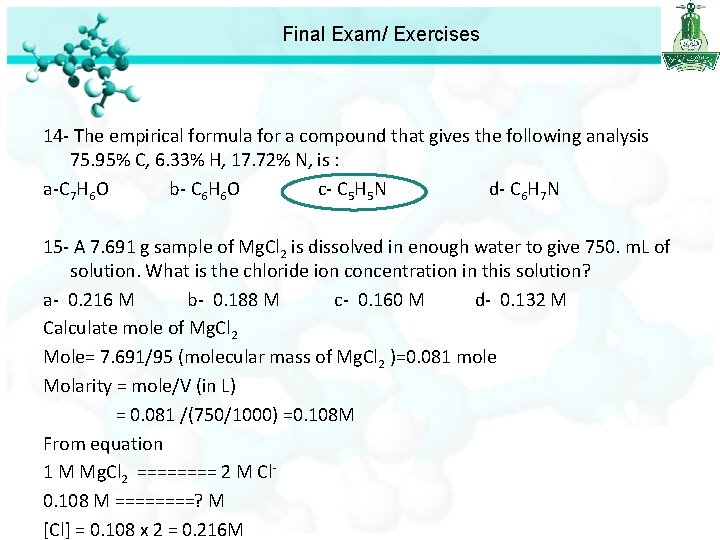Final Exam/ Exercises 14 - The empirical formula for a compound that gives the following analysis 75. 95% C, 6. 33% H, 17. 72% N, is : a-C 7 H 6 O b- C 6 H 6 O c- C 5 H 5 N d- C 6 H 7 N 15 - A 7. 691 g sample of Mg. Cl 2 is dissolved in enough water to give 750. m. L of solution. What is the chloride ion concentration in this solution? a- 0. 216 M b- 0. 188 M c- 0. 160 M d- 0. 132 M Calculate mole of Mg. Cl 2 Mole= 7. 691/95 (molecular mass of Mg. Cl 2 )=0. 081 mole Molarity = mole/V (in L) = 0. 081 /(750/1000) =0. 108 M From equation 1 M Mg. Cl 2 ==== 2 M Cl 0. 108 M ====? M [Cl] = 0. 108 x 2 = 0. 216 M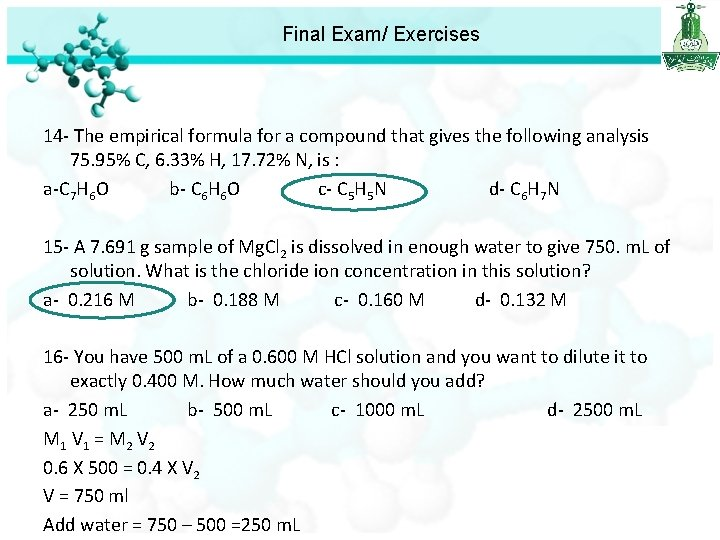Final Exam/ Exercises 14 - The empirical formula for a compound that gives the following analysis 75. 95% C, 6. 33% H, 17. 72% N, is : a-C 7 H 6 O b- C 6 H 6 O c- C 5 H 5 N d- C 6 H 7 N 15 - A 7. 691 g sample of Mg. Cl 2 is dissolved in enough water to give 750. m. L of solution. What is the chloride ion concentration in this solution? a- 0. 216 M b- 0. 188 M c- 0. 160 M d- 0. 132 M 16 - You have 500 m. L of a 0. 600 M HCl solution and you want to dilute it to exactly 0. 400 M. How much water should you add? a- 250 m. L b- 500 m. L c- 1000 m. L d- 2500 m. L M 1 V 1 = M 2 V 2 0. 6 X 500 = 0. 4 X V 2 V = 750 ml Add water = 750 – 500 =250 m. L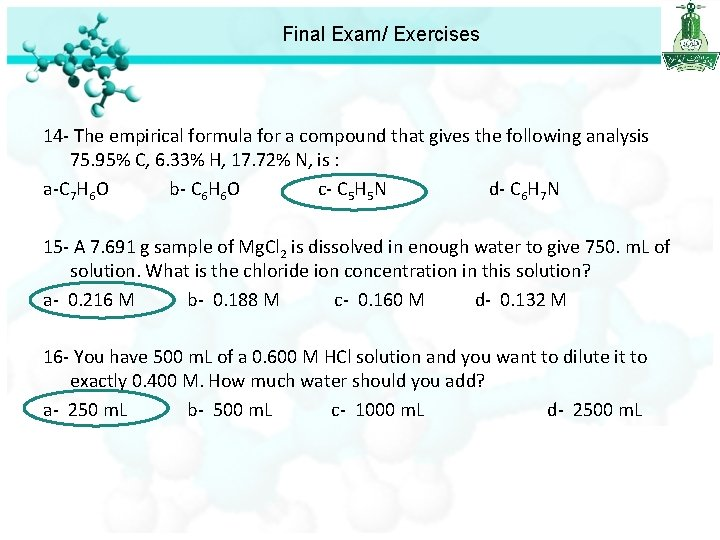Final Exam/ Exercises 14 - The empirical formula for a compound that gives the following analysis 75. 95% C, 6. 33% H, 17. 72% N, is : a-C 7 H 6 O b- C 6 H 6 O c- C 5 H 5 N d- C 6 H 7 N 15 - A 7. 691 g sample of Mg. Cl 2 is dissolved in enough water to give 750. m. L of solution. What is the chloride ion concentration in this solution? a- 0. 216 M b- 0. 188 M c- 0. 160 M d- 0. 132 M 16 - You have 500 m. L of a 0. 600 M HCl solution and you want to dilute it to exactly 0. 400 M. How much water should you add? a- 250 m. L b- 500 m. L c- 1000 m. L d- 2500 m. L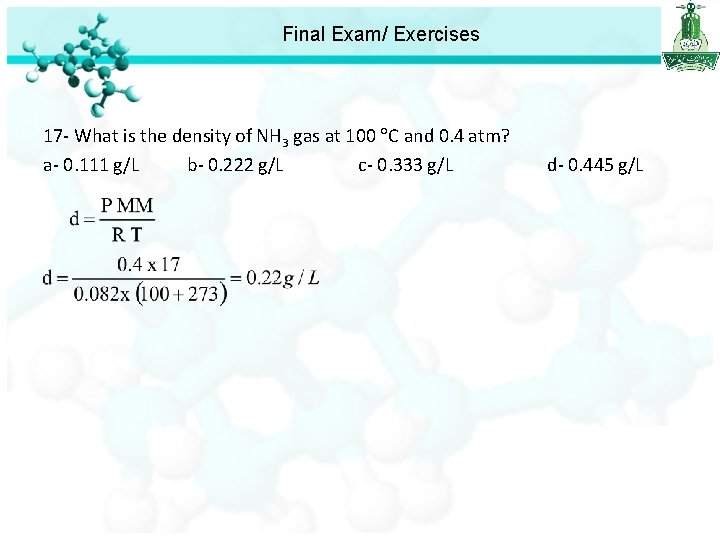Final Exam/ Exercises 17 - What is the density of NH 3 gas at 100 C and 0. 4 atm? a- 0. 111 g/L b- 0. 222 g/L c- 0. 333 g/L d- 0. 445 g/L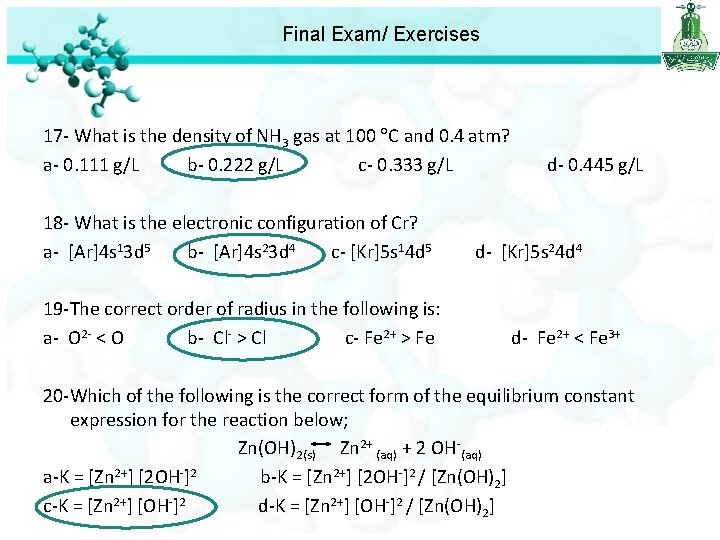Final Exam/ Exercises 17 - What is the density of NH 3 gas at 100 C and 0. 4 atm? a- 0. 111 g/L b- 0. 222 g/L c- 0. 333 g/L d- 0. 445 g/L 18 - What is the electronic configuration of Cr? a- [Ar]4 s 13 d 5 b- [Ar]4 s 23 d 4 c- [Kr]5 s 14 d 5 d- [Kr]5 s 24 d 4 19 -The correct order of radius in the following is: a- O 2 - < O b- Cl- > Cl c- Fe 2+ > Fe d- Fe 2+ < Fe 3+ 20 -Which of the following is the correct form of the equilibrium constant expression for the reaction below; Zn(OH)2(s) Zn 2+ (aq) + 2 OH-(aq) a-K = [Zn 2+] [2 OH-]2 b-K = [Zn 2+] [2 OH-]2 / [Zn(OH)2] c-K = [Zn 2+] [OH-]2 d-K = [Zn 2+] [OH-]2 / [Zn(OH)2]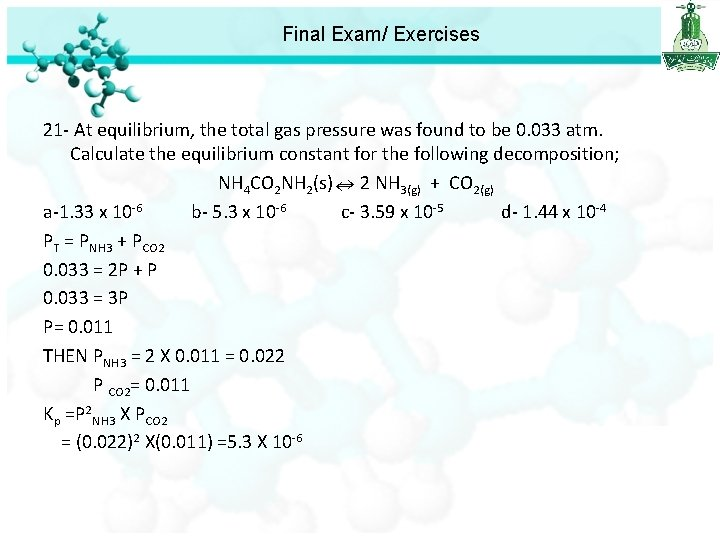Final Exam/ Exercises 21 - At equilibrium, the total gas pressure was found to be 0. 033 atm. Calculate the equilibrium constant for the following decomposition; NH 4 CO 2 NH 2(s) 2 NH 3(g) + CO 2(g) a-1. 33 x 10 -6 b- 5. 3 x 10 -6 c- 3. 59 x 10 -5 d- 1. 44 x 10 -4 PT = PNH 3 + PCO 2 0. 033 = 2 P + P 0. 033 = 3 P P= 0. 011 THEN PNH 3 = 2 X 0. 011 = 0. 022 P CO 2= 0. 011 Kp =P 2 NH 3 X PCO 2 = (0. 022)2 X(0. 011) =5. 3 X 10 -6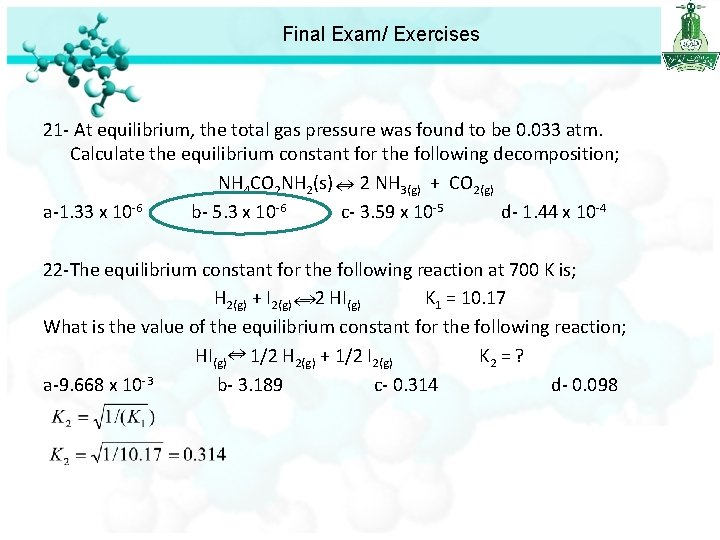Final Exam/ Exercises 21 - At equilibrium, the total gas pressure was found to be 0. 033 atm. Calculate the equilibrium constant for the following decomposition; NH 4 CO 2 NH 2(s) 2 NH 3(g) + CO 2(g) a-1. 33 x 10 -6 b- 5. 3 x 10 -6 c- 3. 59 x 10 -5 d- 1. 44 x 10 -4 22 -The equilibrium constant for the following reaction at 700 K is; H 2(g) + I 2(g) 2 HI (g) K 1 = 10. 17 What is the value of the equilibrium constant for the following reaction; HI(g) 1/2 H 2(g) + 1/2 I 2(g) K 2 = ? a-9. 668 x 10 -3 b- 3. 189 c- 0. 314 d- 0. 098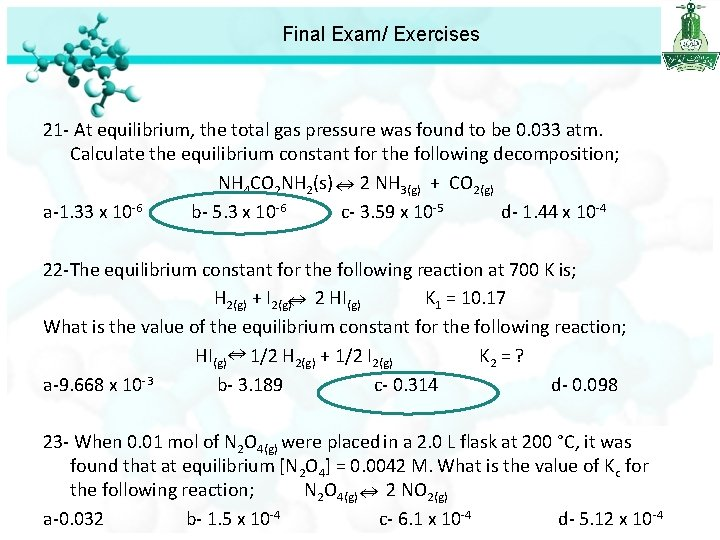Final Exam/ Exercises 21 - At equilibrium, the total gas pressure was found to be 0. 033 atm. Calculate the equilibrium constant for the following decomposition; NH 4 CO 2 NH 2(s) 2 NH 3(g) + CO 2(g) a-1. 33 x 10 -6 b- 5. 3 x 10 -6 c- 3. 59 x 10 -5 d- 1. 44 x 10 -4 22 -The equilibrium constant for the following reaction at 700 K is; H 2(g) + I 2(g) 2 HI(g) K 1 = 10. 17 What is the value of the equilibrium constant for the following reaction; HI(g) 1/2 H 2(g) + 1/2 I 2(g) K 2 = ? a-9. 668 x 10 -3 b- 3. 189 c- 0. 314 d- 0. 098 23 - When 0. 01 mol of N 2 O 4(g) were placed in a 2. 0 L flask at 200 °C, it was found that at equilibrium [N 2 O 4] = 0. 0042 M. What is the value of Kc for the following reaction; N 2 O 4(g) 2 NO 2(g) a-0. 032 b- 1. 5 x 10 -4 c- 6. 1 x 10 -4 d- 5. 12 x 10 -4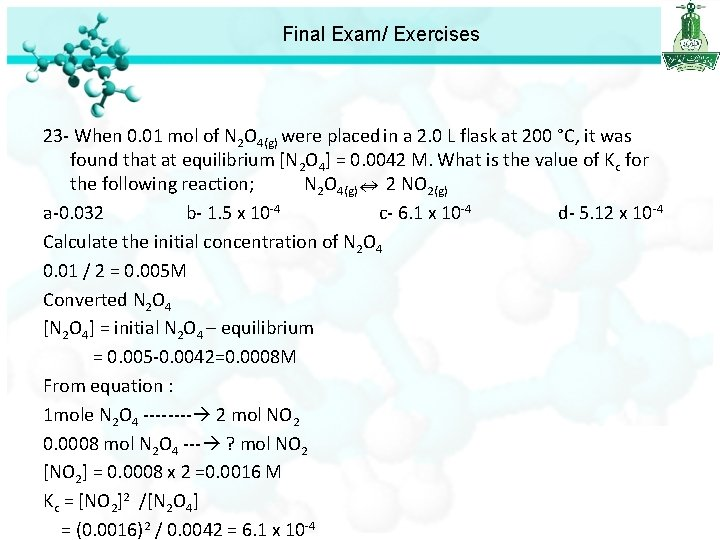Final Exam/ Exercises 23 - When 0. 01 mol of N 2 O 4(g) were placed in a 2. 0 L flask at 200 °C, it was found that at equilibrium [N 2 O 4] = 0. 0042 M. What is the value of Kc for the following reaction; N 2 O 4(g) 2 NO 2(g) a-0. 032 b- 1. 5 x 10 -4 c- 6. 1 x 10 -4 d- 5. 12 x 10 -4 Calculate the initial concentration of N 2 O 4 0. 01 / 2 = 0. 005 M Converted N 2 O 4 [N 2 O 4] = initial N 2 O 4 – equilibrium = 0. 005 -0. 0042=0. 0008 M From equation : 1 mole N 2 O 4 ---- 2 mol NO 2 0. 0008 mol N 2 O 4 --- ? mol NO 2 [NO 2] = 0. 0008 x 2 =0. 0016 M Kc = [NO 2]2 /[N 2 O 4] = (0. 0016)2 / 0. 0042 = 6. 1 x 10 -4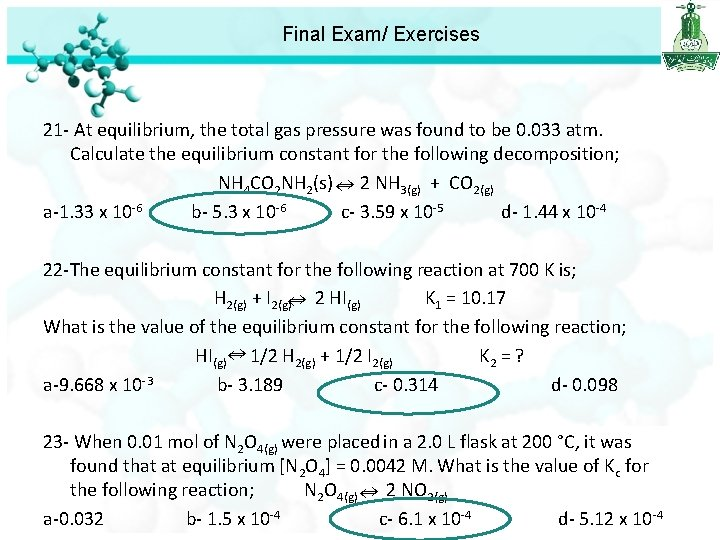Final Exam/ Exercises 21 - At equilibrium, the total gas pressure was found to be 0. 033 atm. Calculate the equilibrium constant for the following decomposition; NH 4 CO 2 NH 2(s) 2 NH 3(g) + CO 2(g) a-1. 33 x 10 -6 b- 5. 3 x 10 -6 c- 3. 59 x 10 -5 d- 1. 44 x 10 -4 22 -The equilibrium constant for the following reaction at 700 K is; H 2(g) + I 2(g) 2 HI(g) K 1 = 10. 17 What is the value of the equilibrium constant for the following reaction; HI(g) 1/2 H 2(g) + 1/2 I 2(g) K 2 = ? a-9. 668 x 10 -3 b- 3. 189 c- 0. 314 d- 0. 098 23 - When 0. 01 mol of N 2 O 4(g) were placed in a 2. 0 L flask at 200 °C, it was found that at equilibrium [N 2 O 4] = 0. 0042 M. What is the value of Kc for the following reaction; N 2 O 4(g) 2 NO 2(g) a-0. 032 b- 1. 5 x 10 -4 c- 6. 1 x 10 -4 d- 5. 12 x 10 -4Final Exam/ Exercises 24 - For the following equilibrium at 25 °C; Cl 2(g) + 3 F 2(g) 2 Cl. F 3(g) If [Cl 2] = 0. 15 M, [F 2] = 0. 28 M and [Cl. F 3] = 0. 01 M at equilibrium, calculate the equilibrium constant Kp for the reaction at the same temperature? a-5. 1 x 10 -5 b- 0. 03 c-18. 13 d- 7. 2 x 10 -3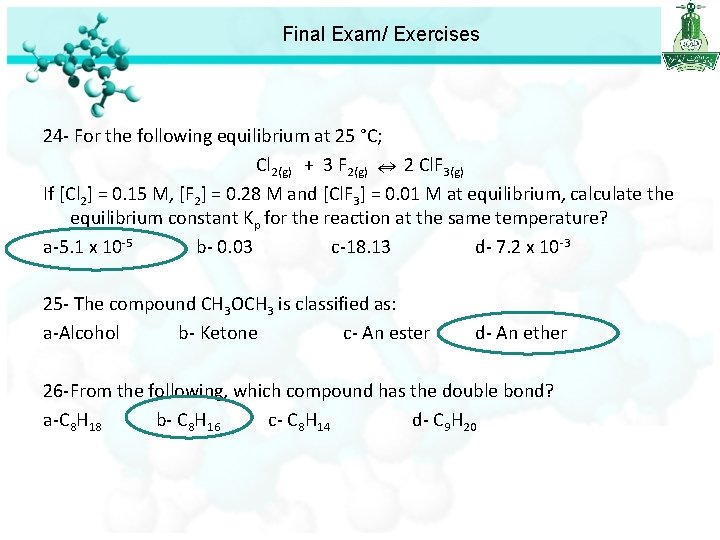Final Exam/ Exercises 24 - For the following equilibrium at 25 °C; Cl 2(g) + 3 F 2(g) 2 Cl. F 3(g) If [Cl 2] = 0. 15 M, [F 2] = 0. 28 M and [Cl. F 3] = 0. 01 M at equilibrium, calculate the equilibrium constant Kp for the reaction at the same temperature? a-5. 1 x 10 -5 b- 0. 03 c-18. 13 d- 7. 2 x 10 -3 25 - The compound CH 3 OCH 3 is classified as: a-Alcohol b- Ketone c- An ester d- An ether 26 -From the following, which compound has the double bond? a-C 8 H 18 b- C 8 H 16 c- C 8 H 14 d- C 9 H 20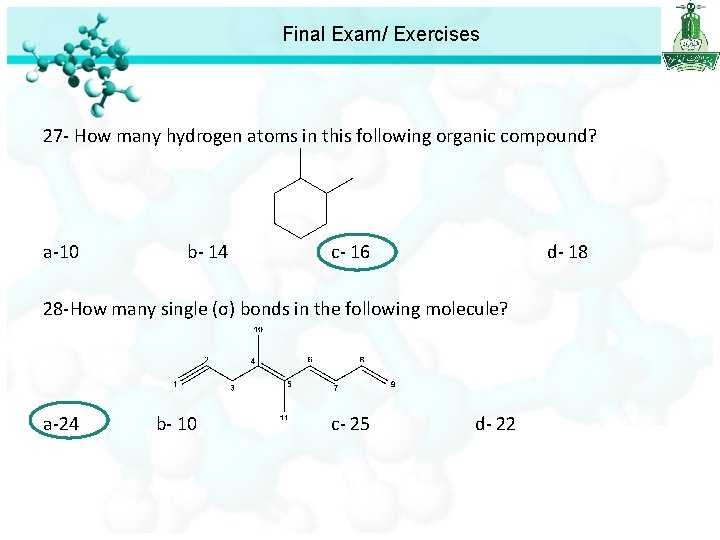Final Exam/ Exercises 27 - How many hydrogen atoms in this following organic compound? a-10 b- 14 c- 16 d- 18 28 -How many single (σ) bonds in the following molecule? a-24 b- 10 c- 25 d- 22Final Exam/ Exercises 29 -From question (28), What is the hybridization for carbon atom number 4 (C 4)? a-sp b- sp 2 c- sp 3 d- sp 3 d 2 30 - The IUPAC name of the following compound is: a-4, 6 -methyl-3 -heptene c- 4, 6 -methyl-3 -heptene b- 4, 6 -dimethyl-4 -heptene d- 4, 6 -dimethyl-3 -heptene 31 - The IUPAC name of the following compound is: a-1, 2, 4 -trichlorobenzene b- 1, 3, 4 -trichlorobenzene c- 1, 2, 3 -trichlorobenzene d- 1, 4, 5 -trichlorobenzeneFinal Exam/ Exercises 32 - What is the concentration of H+ in a 2. 5 M HCl solution? a- 0. 75 M b- 1. 0 M c- 2. 5 M d- 5. 0 M 33 - What is the solubility of Hg. Cl 2 (Ksp = 1. 8 x 10 -18)? a-7. 66 x 10 -7 b- 1. 89 x 10 -3 M c- 7. 8 x 10 -5 M d- 1. 3 x 1 +2 [Hg ]= s [Cl ]= 2 s Ksp = [Hg+2][Cl-]2 Ksp = (s)(2 s)2 Ksp = 4 s 3= 1. 8 x 10 -18 s 3= 4. 5 x 10 -19 s= 7. 66 x 10 -7 M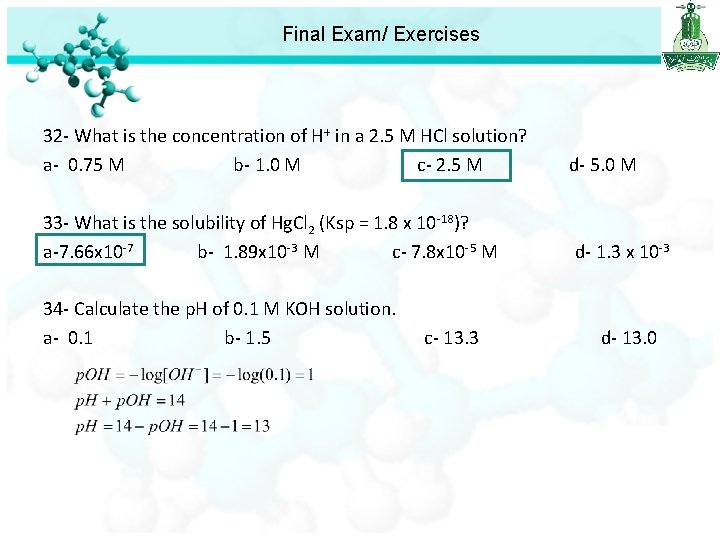Final Exam/ Exercises 32 - What is the concentration of H+ in a 2. 5 M HCl solution? a- 0. 75 M b- 1. 0 M c- 2. 5 M d- 5. 0 M 33 - What is the solubility of Hg. Cl 2 (Ksp = 1. 8 x 10 -18)? a-7. 66 x 10 -7 b- 1. 89 x 10 -3 M c- 7. 8 x 10 -5 M d- 1. 3 x 10 -3 34 - Calculate the p. H of 0. 1 M KOH solution. a- 0. 1 b- 1. 5 c- 13. 3 d- 13. 0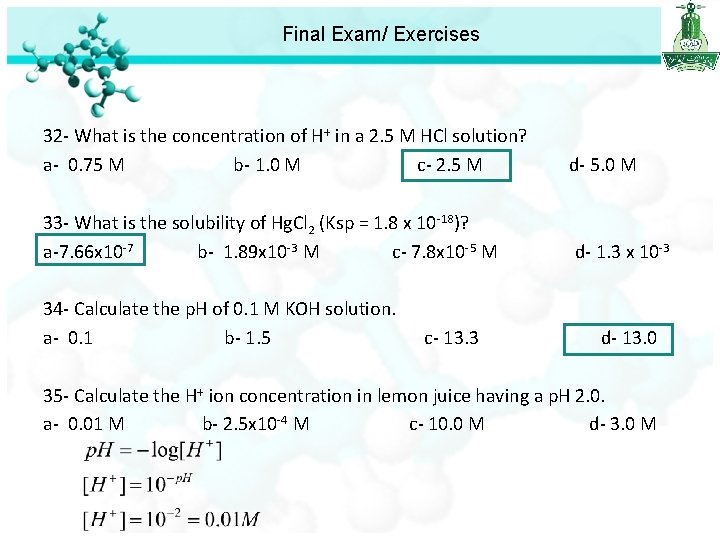Final Exam/ Exercises 32 - What is the concentration of H+ in a 2. 5 M HCl solution? a- 0. 75 M b- 1. 0 M c- 2. 5 M d- 5. 0 M 33 - What is the solubility of Hg. Cl 2 (Ksp = 1. 8 x 10 -18)? a-7. 66 x 10 -7 b- 1. 89 x 10 -3 M c- 7. 8 x 10 -5 M d- 1. 3 x 10 -3 34 - Calculate the p. H of 0. 1 M KOH solution. a- 0. 1 b- 1. 5 c- 13. 3 d- 13. 0 35 - Calculate the H+ ion concentration in lemon juice having a p. H 2. 0. a- 0. 01 M b- 2. 5 x 10 -4 M c- 10. 0 M d- 3. 0 M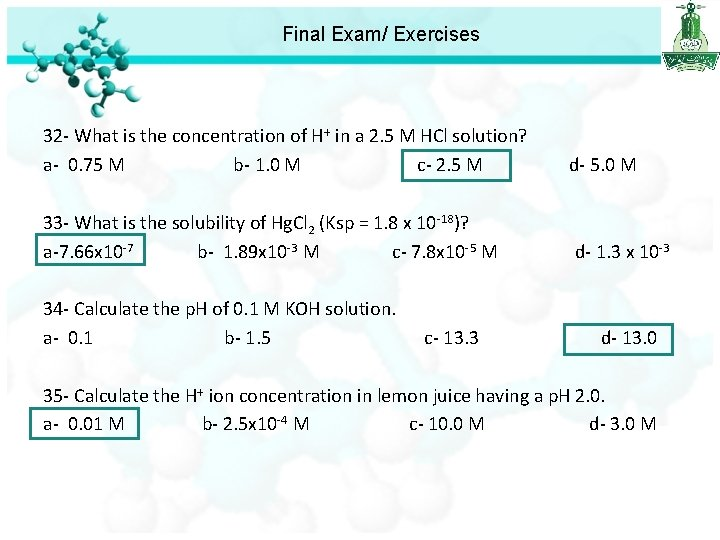Final Exam/ Exercises 32 - What is the concentration of H+ in a 2. 5 M HCl solution? a- 0. 75 M b- 1. 0 M c- 2. 5 M d- 5. 0 M 33 - What is the solubility of Hg. Cl 2 (Ksp = 1. 8 x 10 -18)? a-7. 66 x 10 -7 b- 1. 89 x 10 -3 M c- 7. 8 x 10 -5 M d- 1. 3 x 10 -3 34 - Calculate the p. H of 0. 1 M KOH solution. a- 0. 1 b- 1. 5 c- 13. 3 d- 13. 0 35 - Calculate the H+ ion concentration in lemon juice having a p. H 2. 0. a- 0. 01 M b- 2. 5 x 10 -4 M c- 10. 0 M d- 3. 0 M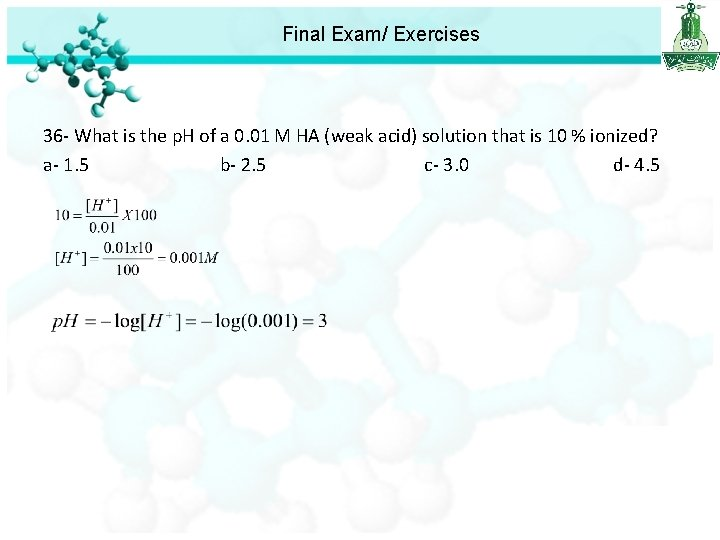Final Exam/ Exercises 36 - What is the p. H of a 0. 01 M HA (weak acid) solution that is 10 % ionized? a- 1. 5 b- 2. 5 c- 3. 0 d- 4. 5Final Exam/ Exercises 36 - What is the p. H of a 0. 01 M HA (weak acid) solution that is 10 % ionized? a- 1. 5 b- 2. 5 c- 3. 0 d- 4. 5 37 - Find the p. H of a 0. 1 M aqueous solution of hypobromous acid (HOBr), for which Ka = 2. 06 10 -9. a- 4. 84 b- 2. 55 c- 3. 0 d- 4. 5 p. H = -log[H+] = 4. 84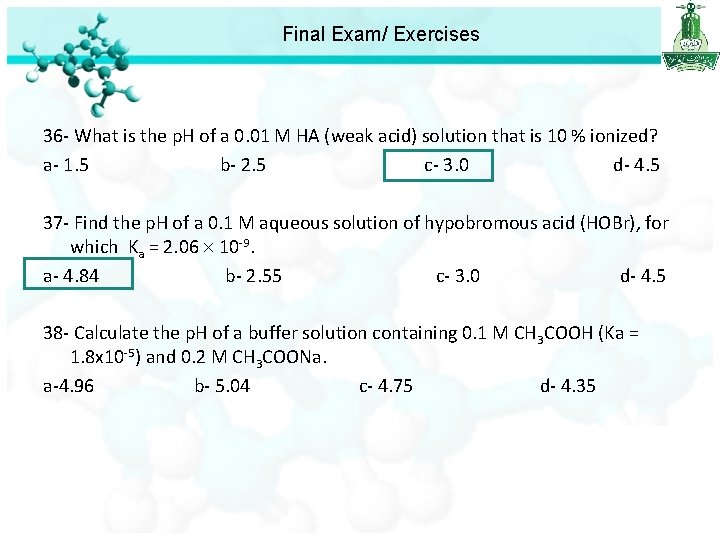Final Exam/ Exercises 36 - What is the p. H of a 0. 01 M HA (weak acid) solution that is 10 % ionized? a- 1. 5 b- 2. 5 c- 3. 0 d- 4. 5 37 - Find the p. H of a 0. 1 M aqueous solution of hypobromous acid (HOBr), for which Ka = 2. 06 10 -9. a- 4. 84 b- 2. 55 c- 3. 0 d- 4. 5 38 - Calculate the p. H of a buffer solution containing 0. 1 M CH 3 COOH (Ka = 1. 8 x 10 -5) and 0. 2 M CH 3 COONa. a-4. 96 b- 5. 04 c- 4. 75 d- 4. 35Final Exam/ Exercises 38 - Calculate the p. H of a buffer solution containing 0. 1 M CH 3 COOH (Ka = 1. 8 x 10 -5) and 0. 2 M CH 3 COONa. a-4. 96 b- 5. 04 c- 4. 75 d- 4. 35 [A-] p. H = p. Ka + log [HA] 0. 2 p. H = -log 1. 8 x 10 -5 +log 0. 1 p. H = 5. 04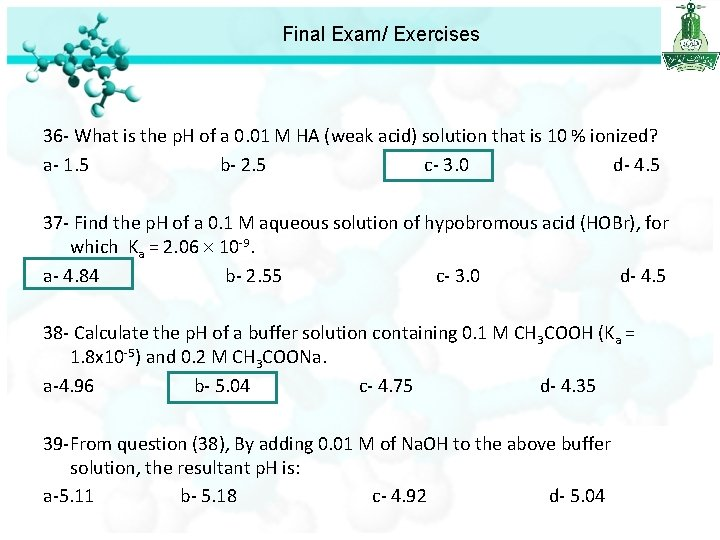Final Exam/ Exercises 36 - What is the p. H of a 0. 01 M HA (weak acid) solution that is 10 % ionized? a- 1. 5 b- 2. 5 c- 3. 0 d- 4. 5 37 - Find the p. H of a 0. 1 M aqueous solution of hypobromous acid (HOBr), for which Ka = 2. 06 10 -9. a- 4. 84 b- 2. 55 c- 3. 0 d- 4. 5 38 - Calculate the p. H of a buffer solution containing 0. 1 M CH 3 COOH (Ka = 1. 8 x 10 -5) and 0. 2 M CH 3 COONa. a-4. 96 b- 5. 04 c- 4. 75 d- 4. 35 39 -From question (38), By adding 0. 01 M of Na. OH to the above buffer solution, the resultant p. H is: a-5. 11 b- 5. 18 c- 4. 92 d- 5. 04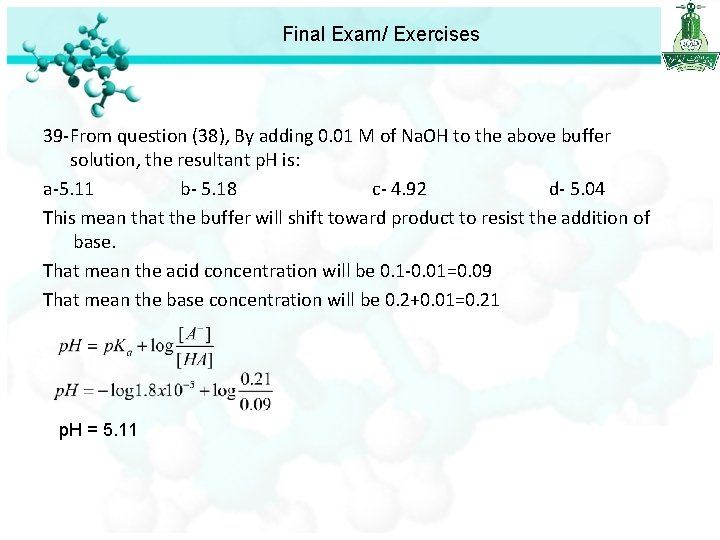Final Exam/ Exercises 39 -From question (38), By adding 0. 01 M of Na. OH to the above buffer solution, the resultant p. H is: a-5. 11 b- 5. 18 c- 4. 92 d- 5. 04 This mean that the buffer will shift toward product to resist the addition of base. That mean the acid concentration will be 0. 1 -0. 01=0. 09 That mean the base concentration will be 0. 2+0. 01=0. 21 p. H = 5. 11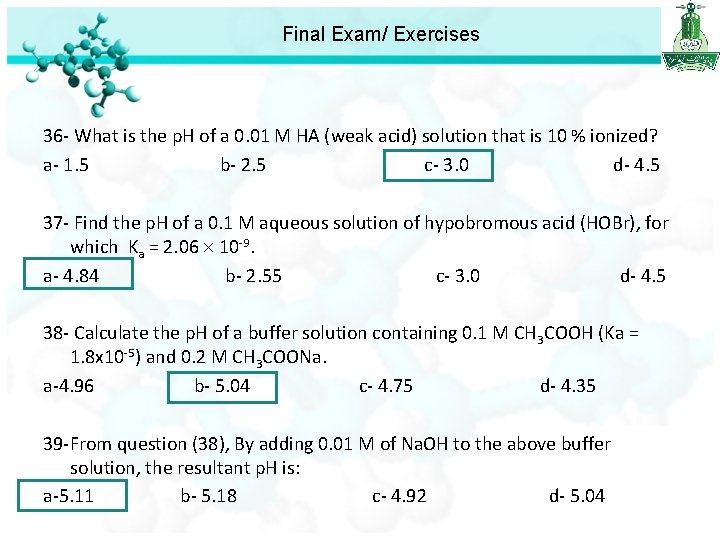Final Exam/ Exercises 36 - What is the p. H of a 0. 01 M HA (weak acid) solution that is 10 % ionized? a- 1. 5 b- 2. 5 c- 3. 0 d- 4. 5 37 - Find the p. H of a 0. 1 M aqueous solution of hypobromous acid (HOBr), for which Ka = 2. 06 10 -9. a- 4. 84 b- 2. 55 c- 3. 0 d- 4. 5 38 - Calculate the p. H of a buffer solution containing 0. 1 M CH 3 COOH (Ka = 1. 8 x 10 -5) and 0. 2 M CH 3 COONa. a-4. 96 b- 5. 04 c- 4. 75 d- 4. 35 39 -From question (38), By adding 0. 01 M of Na. OH to the above buffer solution, the resultant p. H is: a-5. 11 b- 5. 18 c- 4. 92 d- 5. 04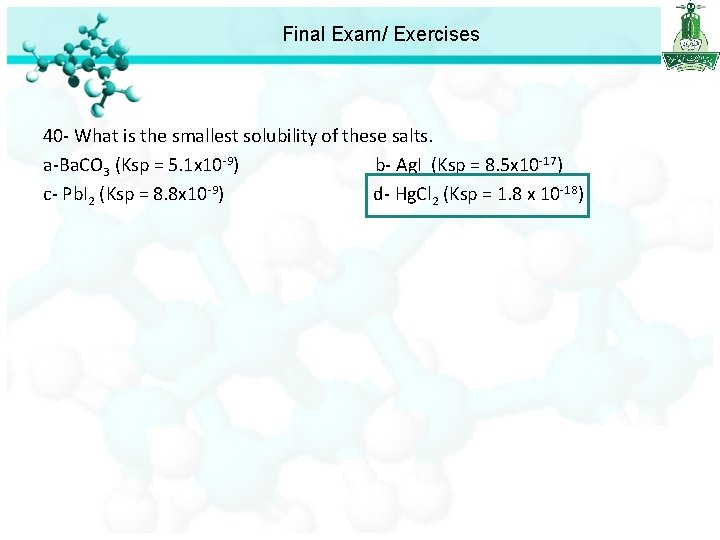Final Exam/ Exercises 40 - What is the smallest solubility of these salts. a-Ba. CO 3 (Ksp = 5. 1 x 10 -9) b- Ag. I (Ksp = 8. 5 x 10 -17) c- Pb. I 2 (Ksp = 8. 8 x 10 -9) d- Hg. Cl 2 (Ksp = 1. 8 x 10 -18)